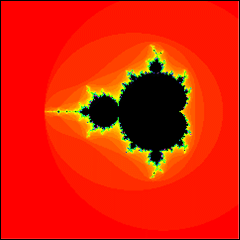##帐号 自动登录 找回密码 密码 欢迎注册
 搜索

# [欣赏] 分形之美发表于 2011-8-28 09:37:03 | 显示全部楼层
 1# G-Spider 请教楼主有三维Hillbert曲线的的分型实现代码吗？自己弄了半天没成功楼主| 发表于 2011-10-21 13:12:28 | 显示全部楼层
 Fractal分形源码一枚：FractalExplorer64.zip (237.81 KB, 下载次数: 5) 2011-10-21 13:12 上传 点击文件名下载附件 效果图：楼主| 发表于 2011-10-21 13:25:31 | 显示全部楼层
 网站分享： http://www.skytopia.com/project/fractal/mandelbulb.html发表于 2012-2-1 18:21:26 | 显示全部楼层
 很美，就是有大图吗？想作为桌面啊发表于 2012-2-11 22:04:10 | 显示全部楼层
 以前也编过，不过没这么漂亮楼主| 发表于 2013-4-2 22:24:23 | 显示全部楼层
 Mandelbulb (3d mandelbrot) Introduction : http://www.skytopia.com/project/fractal/mandelbulb.html Math formula : http://www.skytopia.com/project/fractal/2mandelbulb.html To see the beast : http://www.fractalforums.com/3d- ... uality-mandelbulbs/What's the formula of this thing? There are a few subtle variations, which mostly end up producing the same kind of incredible detail. Listed below is one version. Similar to the original 2D Mandelbrot, the 3D formula is defined by: z -> z^n + c ...but where 'z' and 'c' are hypercomplex ('triplex') numbers, representing Cartesian x, y, and z coordinates. The exponentiation term can be defined by: {x,y,z}^n = r^n { sin(theta*n) * cos(phi*n) , sin(theta*n) * sin(phi*n) , cos(theta*n) } ...where: r = sqrt(x^2 + y^2 + z^2) theta = atan2( sqrt(x^2+y^2), z ) phi = atan2(y,x) And the addition term in z -> z^n + c is similar to standard complex addition, and is simply defined by: {x,y,z}+{a,b,c} = {x+a, y+b, z+c} The rest of the algorithm is similar to the 2D Mandelbrot! Here is some pseudo code of the above: r = sqrt(x*x + y*y + z*z ) theta = atan2(sqrt(x*x + y*y) , z) phi = atan2(y,x) newx = r^n * sin(theta*n) * cos(phi*n) newy = r^n * sin(theta*n) * sin(phi*n) newz = r^n * cos(theta*n) ...where n is the order of the 3D Mandelbulb. Use n=8 to find the exact object in this article. 复制代码http://sourceforge.net/projects/mandelbulber/发表于 2013-4-3 14:42:41 | 显示全部楼层
 用Mathematica做的动态放大Mandelbrot set，另一种配色： mymma.googlecode.com/files/mandel_jet.gif

 您需要登录后才可以回帖 登录 | 欢迎注册 本版积分规则 回帖后跳转到最后一页

GMT+8, 2021-9-27 12:41 , Processed in 0.235303 second(s), 17 queries .